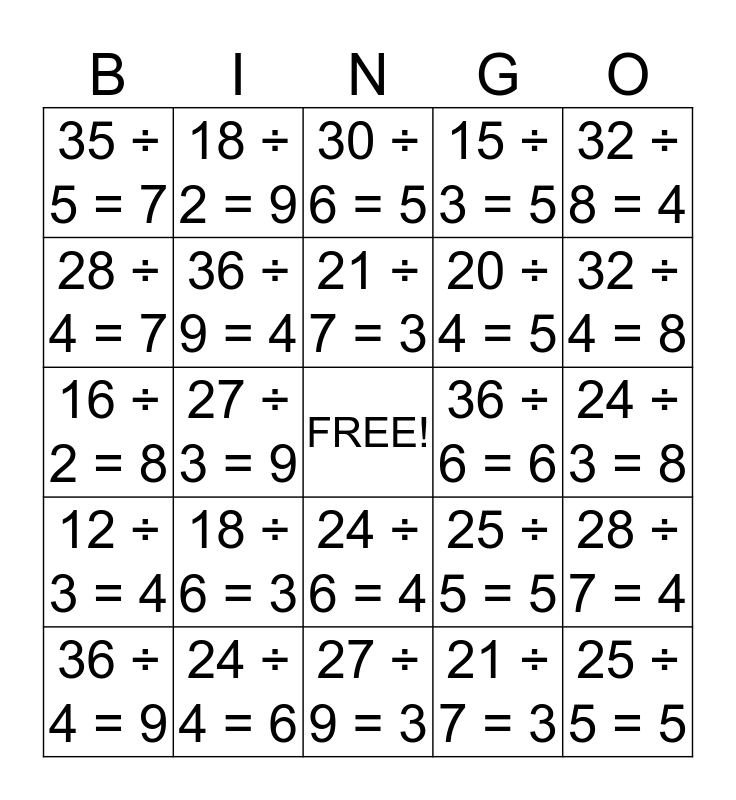# Division BingoThis bingo card has a free space and 24 words: 18 ÷ 6 = 3, 24 ÷ 4 = 6, 21 ÷ 7 = 3, 36 ÷ 4 = 9, 32 ÷ 8 = 4, 35 ÷ 5 = 7, 28 ÷ 4 = 7, 18 ÷ 2 = 9, 36 ÷ 6 = 6, 24 ÷ 6 = 4, 20 ÷ 4 = 5, 16 ÷ 2 = 8, 25 ÷ 5 = 5, 28 ÷ 7 = 4, 36 ÷ 9 = 4, 21 ÷ 7 = 3, 24 ÷ 3 = 8, 30 ÷ 6 = 5, 32 ÷ 4 = 8, 27 ÷ 3 = 9, 25 ÷ 5 = 5, 12 ÷ 3 = 4, 15 ÷ 3 = 5 and 27 ÷ 9 = 3.

⚠ This card has duplicate items: 21 ÷ 7 = 3 (2), 25 ÷ 5 = 5 (2)

## Play Online